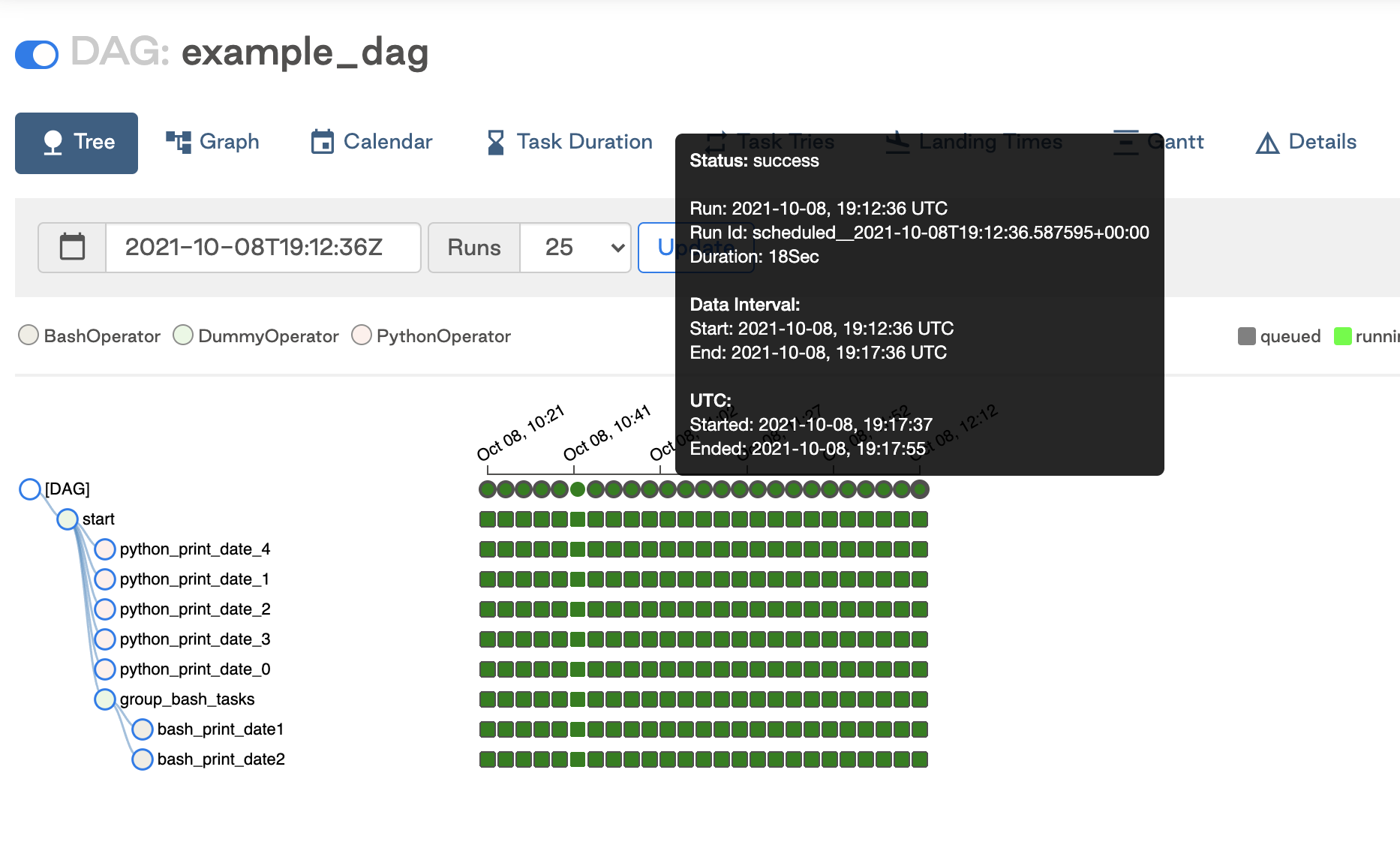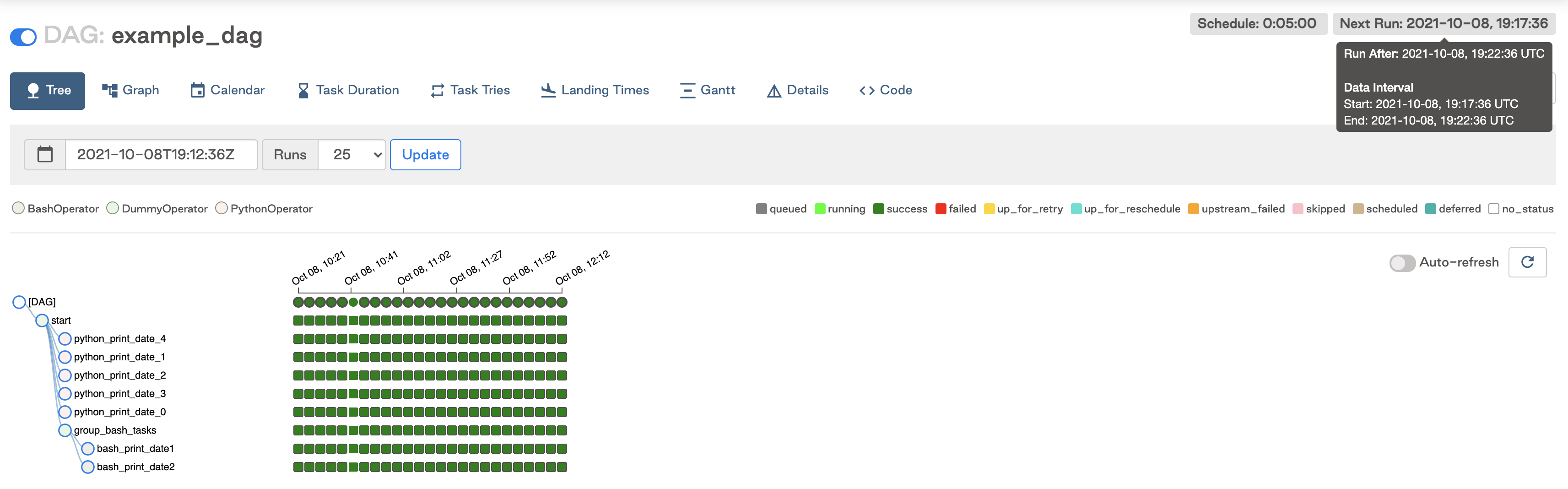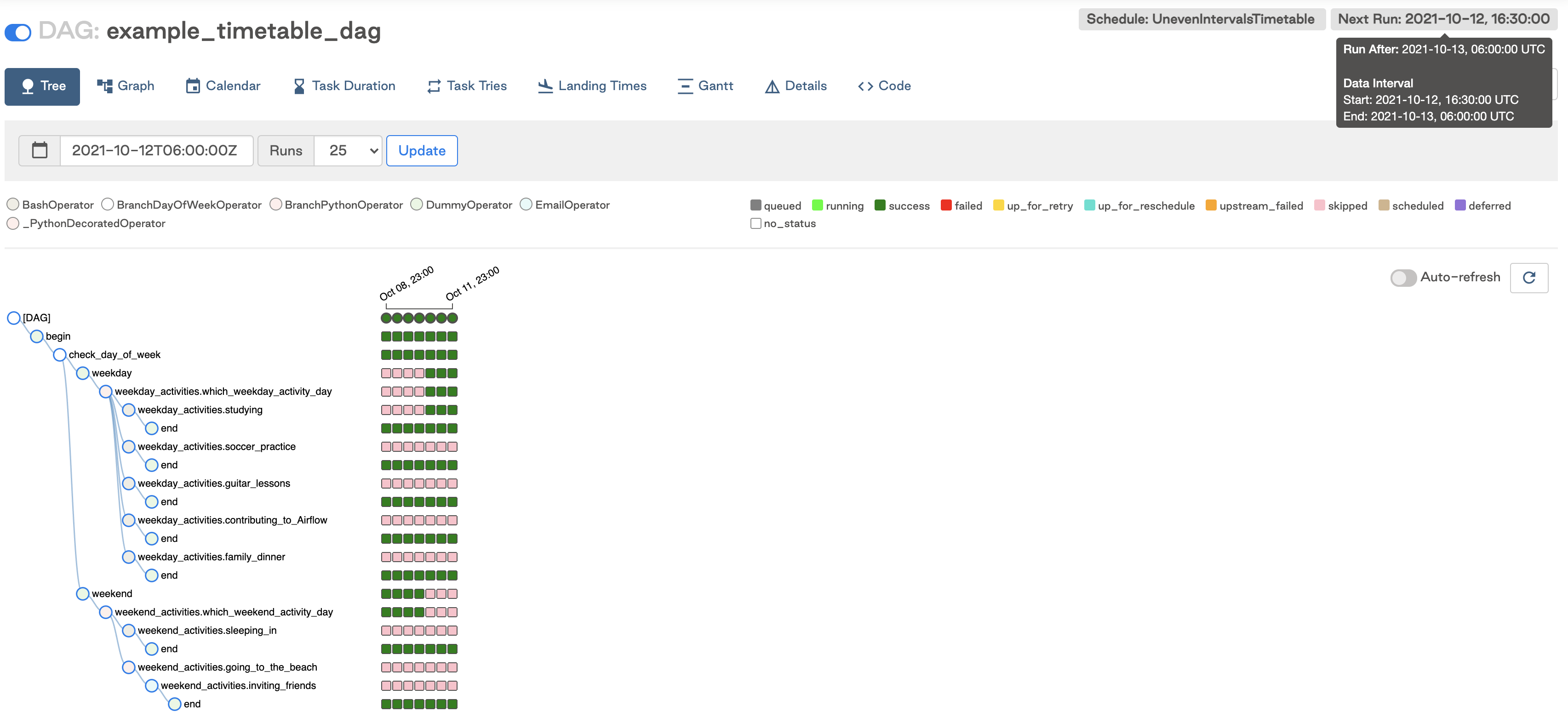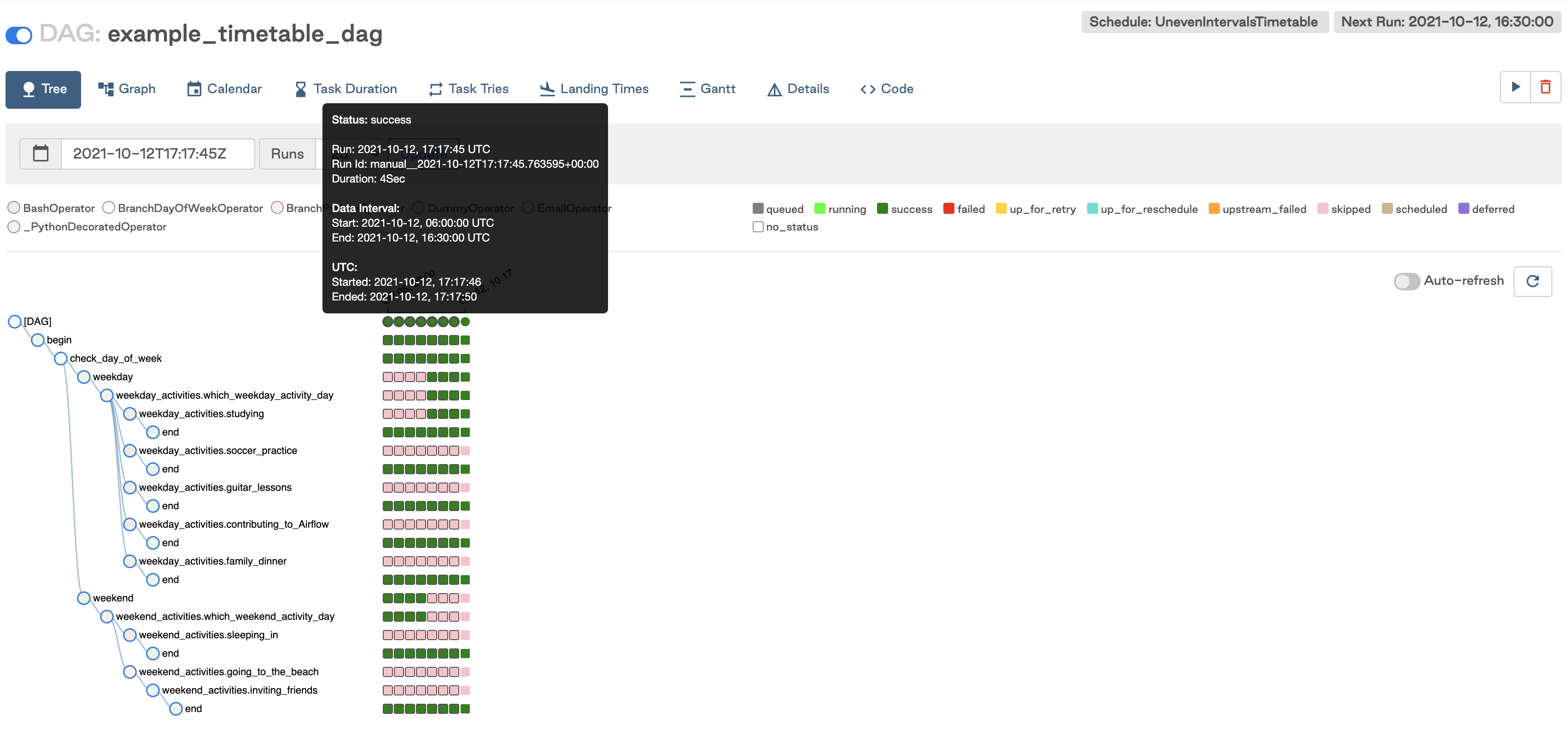View all Guides

Scheduling and Timetables in Airflow

DAGs

Overview

One of the most fundamental features of Apache Airflow is the ability to schedule jobs. Historically, Airflow users could schedule their DAGs by specifying a schedule_interval with a cron expression, a timedelta, or a preset Airflow schedule.

Timetables, released in Airflow 2.2, brought new flexibility to scheduling. Timetables allow users to create their own custom schedules using Python, effectively eliminating the limitations of cron. With timetables, you can now schedule DAGs to run at any time for any use case.

In this guide, we'll walk through Airflow scheduling concepts and the different ways you can schedule a DAG with a focus on timetables. For additional instruction on these concepts, check out our Scheduling in Airflow webinar.

Note: All code in this guide can be found in this repo.

Scheduling Concepts

There are a couple of terms and parameters in Airflow that are important to understand related to scheduling.

Terms

• Data Interval: The data interval is a property of each DAG Run that represents the period of data that each task should operate on. For example, for a DAG scheduled hourly each data interval will begin at the top of the hour (minute 0) and end at the close of the hour (minute 59). The DAG Run is typically executed at the end of the data interval, depending on whether your DAG's schedule has "gaps" in it.
• Logical Date: The logical date of a DAG Run is the same as the start of the data interval. It does not represent when the DAG will actually be executed. Prior to Airflow 2.2, this was referred to as the execution date.
• Timetable: The timetable is a property of a DAG that dictates the data interval and logical date for each DAG Run (i.e. it determines when a DAG will be scheduled).
• Run After: The earliest time the DAG can be scheduled. This date is shown in the Airflow UI, and may be the same as the end of the data interval depending on your DAG's timetable.
• Backfilling and Catchup: We won't cover these concepts in depth here, but they can be related to scheduling. We recommend reading the Apache Airflow documentation on them to understand how they work and whether they're relevant for your use case.

Note: in this guide we do not cover the execution_date concept, which has been deprecated as of Airflow 2.2. If you are using older versions of Airflow, review this doc for more on execution_date.

Parameters

The following parameters are derived from the concepts described above and are important for ensuring your DAG runs at the correct time.

• data_interval_start: A datetime object defining the start date and time of the data interval. A DAG's timetable will return this parameter for each DAG Run. This parameter is either created automatically by Airflow, or can be specified by the user when implementing a custom timetable.
• data_interval_end: A datetime object defining the end date and time of the data interval. A DAG's timetable will return this parameter for each DAG Run. This parameter is either created automatically by Airflow, or can be specified by the user when implementing a custom timetable.
• schedule_interval: A parameter that can be set at the DAG level to define when that DAG will be run. This argument accepts cron expressions or timedelta objects (see the next section for more on this). The schedule_interval can still be defined in Airflow 2.2+, and that schedule will be automatically converted to a timetable by Airflow.
• timetable: A parameter that can be set at the DAG level to define its timetable (either custom or built-in). Timetables can be defined explicitly within the DAG (more on this below), or will be determined automatically by Airflow in cases where a schedule_interval is provided. Either a timetable or a schedule_interval should be defined for each DAG, not both.
• start_date: The first date your DAG will be executed. This parameter is required for your DAG to be scheduled by Airflow.
• end_date: The last date your DAG will be executed. This parameter is optional.

Example

As a simple example of how these concepts work together, say we have a DAG that is scheduled to run every 5 minutes. Taking the most recent DAG Run, the logical date is 2021-10-08 19:12:36, which is the same as the data_interval_start shown in the screenshot below. The data_interval_end is 5 minutes later.If we look at the next run in the UI, the logical date is 2021-10-08 19:17:36. This is 5 minutes after the previous logical date, and the same as the data_interval_end of the last DAG Run since there are no gaps in the schedule. The data interval of the next DAG Run is also shown. Run After, which is the date and time that the next DAG Run is scheduled for, is the same as the current DAG Run's data_interval_end: 2021-10-08 19:22:36.In the sections below, we'll walk through how to use the schedule_interval or timetables to schedule your DAG.

Schedule Interval

For pipelines with basic schedules, you can define a schedule_interval in your DAG. Prior to Airflow 2.2, this was the only mechanism for defining a DAG's schedule.

There are multiple ways you can define the schedule_interval:

Cron expression

You can pass any cron expression as a string to the schedule_interval parameter in your DAG. For example, if you want to schedule your DAG at 4:05 AM every day, you would use schedule_interval='5 4 * * *'.

If you need help creating the correct cron expression, crontab guru is a great resource.

Cron presets

Airflow can utilize cron presets for common, basic schedules.

For example, schedule_interval='@hourly' will schedule the DAG to run at the beginning of every hour. For the full list of presets, check out the Airflow documentation.

Note: If your DAG does not need to run on a schedule and will only be triggered manually or externally triggered by another process, you can set schedule_interval=None.

Timedelta

If you want to schedule your DAG on a particular cadence (hourly, every 5 minutes, etc.) rather than at a specific time, you can pass a timedelta object to the schedule interval. For example, schedule_interval=timedelta(minutes=30) will run the DAG every thirty minutes, and schedule_interval=timedelta(days=1) will run the DAG every day.

Note: Do not make your DAG's schedule dynamic (e.g. datetime.now())! This will cause an error in the Scheduler.

Limitations

The scheduling options detailed in the sections above cover many common use cases, but they do have some limitations. For example, it is difficult or impossible to implement situations like:

• Schedule a DAG at different times on different days, like 2pm on Thursdays and 4pm on Saturdays.
• Schedule a DAG daily except for holidays.
• Schedule a DAG at multiple times daily with uneven intervals (e.g. 1pm and 4:30pm).

In the next section, we'll describe how these limitations were addressed in Airflow 2.2 with the introduction of timetables.

Timetables

Timetables, introduced in Airflow 2.2, address the limitations of cron expressions and timedeltas by allowing users to define their own schedules in Python code. All DAG schedules are determined by their internal timetable.

Going forward, timetables will become the primary method for scheduling in Airflow. You can still define a schedule_interval, but Airflow converts this to a timetable behind the scenes. And if a cron expression or timedelta is not sufficient for your use case, you can define your own timetable.

Custom timetables can be registered as part of an Airflow plugin. They must be a subclass of Timetable, and they should contain the following methods, both of which return a DataInterval with a start and an end:

• next_dagrun_info: Returns the data interval for the DAG's regular schedule
• infer_manual_data_interval: Returns the data interval when the DAG is manually triggered

Below we'll show an example of implementing these methods in a custom timetable.

Example Custom Timetable

For this implementation, let's run our DAG at 6:00 and 16:30. Because this schedule has run times with differing hours and minutes, it can't be represented by a single cron expression. But we can easily implement this schedule with a custom timetable!

To start, we need to define the next_dagrun_info and infer_manual_data_interval methods. Before diving into the code, it's helpful to think through what the data intervals will be for the schedule we want. Remember that the time the DAG runs (run_after) should be the end of the data interval since our interval has no gaps. So in this case, for a DAG that we want to run at 6:00 and 16:30, we have two different alternating intervals:

• Run at 6:00: Data interval is from 16:30 the previous day to 6:00 the current day
• Run at 16:30: Data interval is from 6:00 to 16:30 the current day

With that in mind, first we'll define next_dagrun_info. This method provides Airflow with the logic to calculate the data interval for scheduled runs. It also contains logic to handle the DAG's start_date, end_date, and catchup parameters. To implement the logic in this method, we use the Pendulum package, which makes dealing with dates and times simple. The method looks like this:

def next_dagrun_info(
self,
*,
last_automated_data_interval: Optional[DataInterval],
restriction: TimeRestriction,
) -> Optional[DagRunInfo]:
if last_automated_data_interval is not None:  # There was a previous run on the regular schedule.
last_start = last_automated_data_interval.start
delta = timedelta(days=1)
if last_start.hour == 6: # If previous period started at 6:00, next period will start at 16:30 and end at 6:00 following day
next_start = last_start.set(hour=16, minute=30).replace(tzinfo=UTC)
next_end = (last_start+delta).replace(tzinfo=UTC)
else: # If previous period started at 14:30, next period will start at 6:00 next day and end at 14:30
next_start = (last_start+delta).set(hour=6, minute=0).replace(tzinfo=UTC)
next_end = (last_start+delta).replace(tzinfo=UTC)
else:  # This is the first ever run on the regular schedule. First data interval will always start at 6:00 and end at 16:30
next_start = restriction.earliest
if next_start is None:  # No start_date. Don't schedule.
return None
if not restriction.catchup: # If the DAG has catchup=False, today is the earliest to consider.
next_start = max(next_start, DateTime.combine(Date.today(), Time.min).replace(tzinfo=UTC))
next_start = next_start.set(hour=6, minute=0).replace(tzinfo=UTC)
next_end = next_start.set(hour=16, minute=30).replace(tzinfo=UTC)
if restriction.latest is not None and next_start > restriction.latest:
return None  # Over the DAG's scheduled end; don't schedule.
return DagRunInfo.interval(start=next_start, end=next_end)

Walking through the logic, this code is equivalent to:

• If there was a previous run for the DAG:

• If the previous DAG Run started at 6:00, then the next DAG run should start at 16:30 and end at 6:00 the next day.
• If the previous DAG run started at 16:30, then the DAG run should start at 6:00 the next day and end at 16:30 the next day.
• If it is the first run of the DAG:

• Check for a start date. If there isn't one, the DAG can't be scheduled.
• Check if catchup=False. If so, the earliest date to consider should be the current date. Otherwise it is the DAG's start date.
• We're mandating that the first DAG Run should always start at 6:00, so update the time of the interval start to 6:00 and the end to 16:30.
• If the DAG has an end date, do not schedule the DAG after that date has passed.

Then we define the data interval for manually triggered DAG Runs by defining the infer_manual_data_interval method. The code looks like this:

def infer_manual_data_interval(self, run_after: DateTime) -> DataInterval:
delta = timedelta(days=1)
# If time is between 6:00 and 16:30, period ends at 6am and starts at 16:30 previous day
if run_after >= run_after.set(hour=6, minute=0) and run_after <= run_after.set(hour=16, minute=30):
start = (run_after-delta).set(hour=16, minute=30, second=0).replace(tzinfo=UTC)
end = run_after.set(hour=6, minute=0, second=0).replace(tzinfo=UTC)
# If time is after 16:30 but before midnight, period is between 6:00 and 16:30 the same day
elif run_after >= run_after.set(hour=16, minute=30) and run_after.hour <= 23:
start = run_after.set(hour=6, minute=0, second=0).replace(tzinfo=UTC)
end = run_after.set(hour=16, minute=30, second=0).replace(tzinfo=UTC)
# If time is after midnight but before 6:00, period is between 6:00 and 16:30 the previous day
else:
start = (run_after-delta).set(hour=6, minute=0).replace(tzinfo=UTC)
end = (run_after-delta).set(hour=16, minute=30).replace(tzinfo=UTC)
return DataInterval(start=start, end=end)

This method figures out what the most recent complete data interval is based on the current time. There are three scenarios:

• The current time is between 6:00 and 16:30: In this case, the data interval is from 16:30 the previous day to 6:00 the current day.
• The current time is after 16:30 but before midnight: In this case, the data interval is from 6:00 to 16:30 the current day.
• The current time is after midnight but before 6:00: In this case, the data interval is from 6:00 to 16:30 the previous day.

We need to account for time periods in the same timeframe (6:00 to 16:30) on different days than the day that the DAG is triggered, which requires three sets of logic. When defining custom timetables, always keep in mind what the last complete data interval should be based on when the DAG should run.

Now we can take those two methods and combine them into a Timetable class which will make up our Airflow plugin. The full custom timetable plugin is below:

from datetime import timedelta
from typing import Optional
from pendulum import Date, DateTime, Time, timezone

from airflow.plugins_manager import AirflowPlugin
from airflow.timetables.base import DagRunInfo, DataInterval, TimeRestriction, Timetable

UTC = timezone("UTC")

class UnevenIntervalsTimetable(Timetable):

def infer_manual_data_interval(self, run_after: DateTime) -> DataInterval:
delta = timedelta(days=1)
# If time is between 6:00 and 16:30, period ends at 6am and starts at 16:30 previous day
if run_after >= run_after.set(hour=6, minute=0) and run_after <= run_after.set(hour=16, minute=30):
start = (run_after-delta).set(hour=16, minute=30, second=0).replace(tzinfo=UTC)
end = run_after.set(hour=6, minute=0, second=0).replace(tzinfo=UTC)
# If time is after 16:30 but before midnight, period is between 6:00 and 16:30 the same day
elif run_after >= run_after.set(hour=16, minute=30) and run_after.hour <= 23:
start = run_after.set(hour=6, minute=0, second=0).replace(tzinfo=UTC)
end = run_after.set(hour=16, minute=30, second=0).replace(tzinfo=UTC)
# If time is after midnight but before 6:00, period is between 6:00 and 16:30 the previous day
else:
start = (run_after-delta).set(hour=6, minute=0).replace(tzinfo=UTC)
end = (run_after-delta).set(hour=16, minute=30).replace(tzinfo=UTC)
return DataInterval(start=start, end=end)

def next_dagrun_info(
self,
*,
last_automated_data_interval: Optional[DataInterval],
restriction: TimeRestriction,
) -> Optional[DagRunInfo]:
if last_automated_data_interval is not None:  # There was a previous run on the regular schedule.
last_start = last_automated_data_interval.start
delta = timedelta(days=1)
if last_start.hour == 6: # If previous period started at 6:00, next period will start at 16:30 and end at 6:00 following day
next_start = last_start.set(hour=16, minute=30).replace(tzinfo=UTC)
next_end = (last_start+delta).replace(tzinfo=UTC)
else: # If previous period started at 14:30, next period will start at 6:00 next day and end at 14:30
next_start = (last_start+delta).set(hour=6, minute=0).replace(tzinfo=UTC)
next_end = (last_start+delta).replace(tzinfo=UTC)
else:  # This is the first ever run on the regular schedule. First data interval will always start at 6:00 and end at 16:30
next_start = restriction.earliest
if next_start is None:  # No start_date. Don't schedule.
return None
if not restriction.catchup: # If the DAG has catchup=False, today is the earliest to consider.
next_start = max(next_start, DateTime.combine(Date.today(), Time.min).replace(tzinfo=UTC))
next_start = next_start.set(hour=6, minute=0).replace(tzinfo=UTC)
next_end = next_start.set(hour=16, minute=30).replace(tzinfo=UTC)
if restriction.latest is not None and next_start > restriction.latest:
return None  # Over the DAG's scheduled end; don't schedule.
return DagRunInfo.interval(start=next_start, end=next_end)

class UnevenIntervalsTimetablePlugin(AirflowPlugin):
name = "uneven_intervals_timetable_plugin"
timetables = [UnevenIntervalsTimetable]

Note that because timetables are plugins, you will need to restart the Airflow Scheduler and Webserver after adding or updating them.

In the DAG, we can then import the custom timetable plugin and use it to schedule the DAG by setting the timetable parameter:

from uneven_intervals_timetable import UnevenIntervalsTimetable

with DAG(
dag_id="example_timetable_dag",
start_date=datetime(2021, 10, 9),
max_active_runs=1,
timetable=UnevenIntervalsTimetable(),
default_args={
"retries": 1,
"retry_delay": timedelta(minutes=3),
},
catchup=True
) as dag:

Looking at the Tree View in the UI, we can see that this DAG has run twice per day at 6:00 and 16:30 since the start date of 10/9/2021.The next scheduled run is for the interval starting on 10/12/2021 at 16:30 and ending the following day at 6:00. This run will be triggered at the end of the data interval, so after 10/13/2021 6:00.If we run the DAG manually after 16:30 but before midnight, we can see the data interval for the triggered run was between 6:00 and 16:30 that day as expected.This is a simple timetable that could easily be adjusted to suit other use cases. In general, timetables are completely customizable as long as the methods above are implemented.

Note: Be careful when implementing your timetable logic that your next_dagrun_info method does not return a data_interval_start that is earlier than your DAG's start_date. This will result in tasks not being executed for that DAG Run.

Current Limitations

There are some limitations to keep in mind when implementing custom timetables:

• Timetable methods should return the same result every time they are called (e.g. avoid things like HTTP requests). They are not designed to implement event-based triggering.
• Timetables are parsed by the scheduler when creating DagRuns, so avoid slow or lengthy code that could impact Airflow performance.